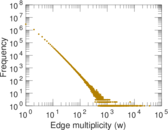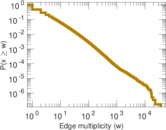Discogs artist–style

Discogs (short for "discographies") is a large online music database that provides information about audio records including information about artists, labels and release details. Each edge of this bipartite network connects an artist and a style. An edge indicates that the artist was involved in the production of a release of the style. Releases can have multiple styles. The left nodes represent artists and the right nodes represent styles.

 Code Dt Internal name discogs_style Name Discogs artist–style Data source http://www.discogs.com/ AvailabilityDataset is available for download Consistency checkDataset passed all tests Category Feature network Node meaning Artist, style Edge meaning Style Network formatBipartite, undirected Edge typeUnweighted, multiple edges

Statistics

 Size n = 1,618,326 Left size n1 = 1,617,943 Right size n2 = 383 Volume m = 24,085,580 Unique edge count m̿ = 5,740,842 Wedge count s = 166,393,512,911 Claw count z = 5,367,492,614,640,449 Cross count x = 1.617 65 × 1020 Square count q = 77,383,418,076 4-Tour count T4 = 1,284,657,683,652 Maximum degree dmax = 1,109,229 Maximum left degree d1max = 621,250 Maximum right degree d2max = 1,109,229 Average degree d = 29.766 0 Average left degree d1 = 14.886 5 Average right degree d2 = 62,886.6 Fill p = 0.009 264 32 Average edge multiplicity m̃ = 4.195 48 Size of LCC N = 1,618,326 Diameter δ = 6 50-Percentile effective diameter δ0.5 = 3.452 57 90-Percentile effective diameter δ0.9 = 3.890 52 Median distance δM = 4 Mean distance δm = 3.827 03 Gini coefficient G = 0.894 557 Balanced inequality ratio P = 0.119 342 Left balanced inequality ratio P1 = 0.181 377 Right balanced inequality ratio P2 = 0.198 666 Relative edge distribution entropy Her = 0.707 633 Power law exponent γ = 2.161 07 Tail power law exponent γt = 2.891 00 Degree assortativity ρ = −0.100 821 Degree assortativity p-value pρ = 0.000 00 Spectral norm α = 89,274.1 Spectral separation |λ1[A] / λ2[A]| = 4.222 26 Controllability C = 1,617,560 Relative controllability Cr = 0.999 527

Plots

Degree distribution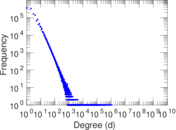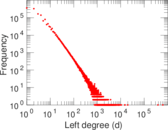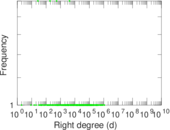Cumulative degree distribution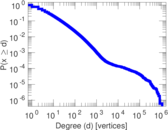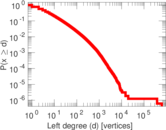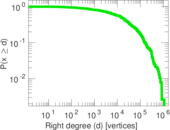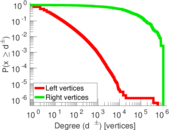Lorenz curve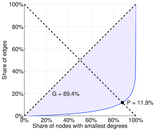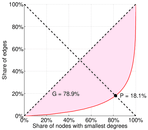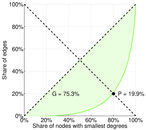Spectral distribution of the adjacency matrix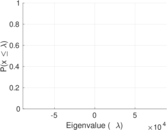Spectral distribution of the normalized adjacency matrix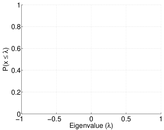Spectral distribution of the Laplacian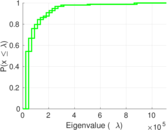Spectral graph drawing based on the adjacency matrix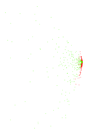Spectral graph drawing based on the Laplacian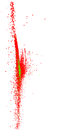Spectral graph drawing based on the normalized adjacency matrix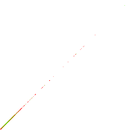Zipf plot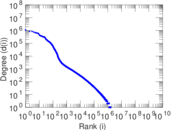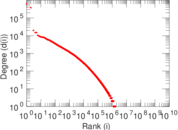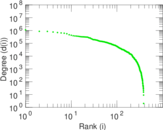Hop distribution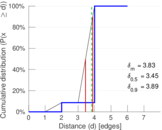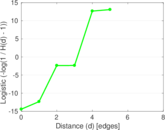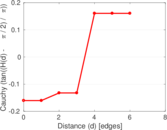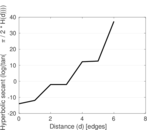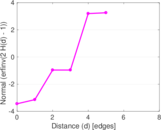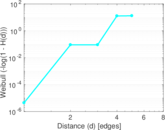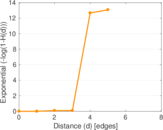Delaunay graph drawing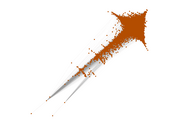Edge weight/multiplicity distribution